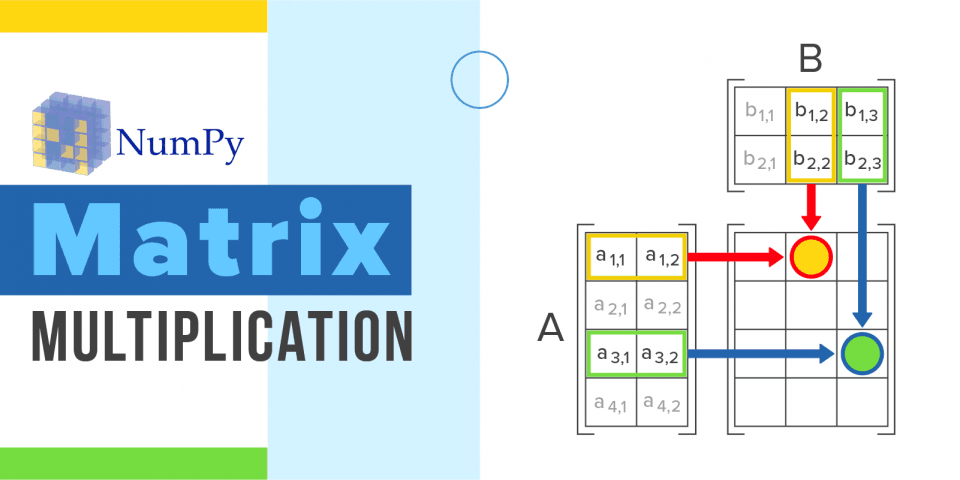Need a discount on popular programming courses? Find them here. View offers

## Python

Disclosure: Hackr.io is supported by its audience. When you purchase through links on our site, we may earn an affiliate commission.

# Numpy Matrix Multiplication

Posted in PythonNumPy, also known as Numerical Python, was created by Travis Oliphant, accomplished by blending the features of Numarray into a Numeric package. NumPy has acted as a “replacement” for Matlab (used for technical computing) in the past; How? The combination of NumPy with packages like SciPy (known as Scientific Python) and Mat−plotlib (plotting library), has been treated as a Python Alternative to Matlab, thus being observed as a more modern and organized programming language. Since NumPy is open-source, it is an extra advantage for programming aspirants and experienced developers.

## What is a NumPy array?

NumPy arrays are similar to Python lists. The NumPy library provides an array of data structure that holds some benefits over Python lists, like--faster access in reading and writing items, is more compact, and is more convenient and efficient.

NumPy is known to provide access to a few substantial tools and techniques that can be utilized to solve mathematical models of problems, that primarily belong to the complexity offered by Science and Engineering. An example, one of these tools is a high-performance multidimensional array object--a robust data structure, best used for efficient computation of arrays and matrices. To operate and function, the best of these arrays requires credibility to solve high-level mathematical functions.

Few categories of necessary array manipulations are listed below:

Attributes of arrays: Determining the size, shape, data types and memory consumption of arrays

1. Mathematical and other logical operations on arrays.
2. Reshaping of arrays: Altering the shape of a provided array.
3. Routines and Fourier transform for shape manipulation.
4. Linear Algebra Operations.
5. Slicing of arrays: Setting up smaller subarrays within a given larger array.
6. Splitting and Joining of arrays: Splitting one array into many and combining multiple arrays into one single array.
7. Indexing of arrays: Setting the value of individual array elements.

### How to Install NumPy:

PC easily installs NumPy by following these simple steps:

#### With Python Wheels:

2. If you are using Windows, add Python to the PATH environment variable.
3. Install a package manager, such as pip (done to ensure that you can use Python’s open-source libraries.

#### NumPy Multiplication Matrix

For multiplying two matrices, use the dot () method. Here is an introduction to numpy.dot( a, b, out=None)

Few specifications of numpy.dot:

• If both a and b are 1-D (one dimensional) arrays -- Inner product of two vectors (without complex conjugation)
• If both a and b are 2-D (two dimensional) arrays -- Matrix multiplication
• If either a or b is 0-D (also known as a scalar) -- Multiply by using numpy.multiply(a, b) or a * b.
• If a is an N-D array and b is a 1-D array -- Sum product over the last axis of a and b.
• If a is an N-D array and b is an M-D array provided that M>=2 -- Sum product over the last axis of a and the second-to-last axis of b:

Also, dot(a, b)[i,j,k,m] = sum(a[i,j,:] * b[k,:,m])

The following code is used to produce a Numpy Multiplication Matrix; * is used for array multiplication.## Summary

This is complete brief about numpy matrix multiplication. Being a great alternative to Python Lists, NumPy arrays are fast and are easier to work. Users have the opportunity to perform calculations across entire arrays, with NumPy, and get fancy with their programs.

Since NumPy arrays occupy less memory as compared to a list, it allows better ways of handling data for Mathematical Operations. There’s a reason why the analytic community favours NumPy array, give it a try.#### Shruti Kaushik

Shruti is a professionally accredited content specialist and works closely with brands to identify their disconnect in content marketing, then further strategizing the same. She started pursuing her independent journey as a consultant after leaving her decent 9 to 5 job with Google News as an editor, and have worked withSony, Ministry of Skills and Entrepreneurship Ma Foi Group, TOI, Indochine International, Kakaku, Inc in the past. View all posts by the Author